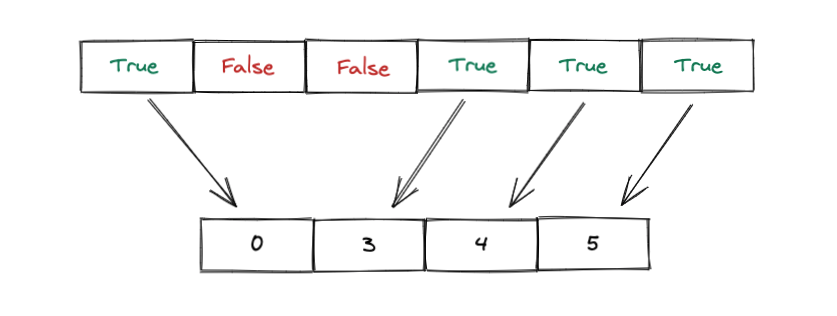Using a Subset of data in PyTorch

When training deep learning models, you'll often want to try out new ideas and see what effect it has on your model.

It becomes very important to have a very high iteration speed. The faster you can train a model, the faster you can test different ideas and see how they impact the performance of the model.

The more experiments you can do, the better!
-- Deep Learning for Coders with fastai & PyTorch

If your model takes too long to train, you can reduce the training time by either using a simpler model, or by using a smaller dataset.

One way to reduce the size of a dataset is to use only a subset of the classes it contains. The Imagenette dataset is an example of this. It contains a subset of 10 classes from the larger ImageNet dataset. Because it's smaller in size, it allows anyone to train state-of-the-art image classification models even if they don't have access to state-of-the-art computing resources, in a short period of time.

In this short post, we'll learn how to use the Subset class in PyTorch to use a small part of a larger dataset for training models quickly.

The method we will learn applies to any instance of a PyTorch dataset. For simplicity, let us assume we are interested in using the CIFAR10 dataset.

# import the required modules
import torch
from torchvision.datasets import CIFAR10
from torchvision import transforms

# No fancy transforms, we just convert the image to a tensor
transform = transforms.ToTensor()

# create training dataset

Let us assume that we want to create a subset with just two classes from this complete dataset: 1 and 8.

The first thing we would need to do is get the index of all samples in this dataset that have classes 1 and 8.

# We create a tensor that has True at an index if the sample belongs to class 1
idx1 = torch.tensor(trainset.targets) == 1

# Similarly, this tensor has True at an index if the sample belongs to class 8
idx8 = torch.tensor(trainset.targets) == 8

We then merge these two so that we have one Boolean tensor that has True at the index where the sample is of class 1 or 8, and False otherwise.

tensor([False, False, False,  ..., False,  True,  True])

We used the bitwise OR operator here.

In a nutshell, this operator gives us an output of False at a particular index if items in idx1 and idx8 at that index are BOTH False.

If either idx1 or idx8 have True at that index (which means the sample at that index is of either class 1 or class 8), then the tensor will have a value of True at that index.We then need to convert this into a list of indices at which we have True.We can do this using the nonzero method in PyTorch.

train_indices
tensor([    4,     5,     8,  ..., 49993, 49998, 49999])

We can then create a subset by specifying these indices as follows:

# First, we import the Subset class
from torch.utils.data import Subset

# We then pass the original dataset and the indices we are interested in
train_subset = Subset(trainset, train_indices)

The subset will now only pick samples from the underlying dataset at the indices which have a value of True in the train_indices that we passed.

We can then use train_subset like any other dataset in PyTorch.

Let us create a DataLoader with the subset and verify it fetches only samples of the classes we have specified.

# Create a dataloader from the subset as usual